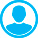AFFINOR METRIC STRUCTURES AND THEIR PHYSICAL APPLICATIONSAbstract (English):
This work describes the fundamentals of the mathematical theory of affinor metric structures and physical problems where these structures are used. Affinor metric structure is defined as an arbitrary 1-form having a radical of arbitrary rank, a certain Riemannian metric and a special field of automorphisms of tangent spaces connecting the exterior differential of this 1-form and a metric. Affinor metric structures are a generalization of almost contact metric structures and Kahlerian structures with a precise fundamental 2-form. At the end of the work, applications of affinor metric structures in physics are described. The purpose of this article is to demonstrate possibilities of application of the theory of affinor metric structures when solving various mathematical and physical problems. In particular, the use of such structures to search for closed curves with a nonzero circulation of a vector field and construction of submanifolds on which the exterior differential of some 1-form induced by a vector field is non-degenerated. Methods of Riemann geometry and theories of differential forms and mathematical analysis on manifolds were used. The relevance of the subject matter is due to the most common case of statement of the problem for some 1-form with radical of arbitrary dimension. While in classic case, physics and geometry consider only 1-forms with a zero radical.

Keywords:
Affinor metric structure, radical of a 1-form, vector field effect on a curve